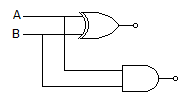# Electronics and Communication Engineering - Digital Electronics

11.

In 8085 microprocessor, how many I/O ports can be accessed by direct method?

 A. 8 B. 256 C. 32 K D. 64 K

Explanation:

No answer description available for this question. Let us discuss.

12.

If the mantissa of a digital computer is 37 bit long then the accuracy of the digital computer will be of

 A. 37 decimal places B. 23 decimal places C. 11 decimal places D. accuracy is independent of the length to mantissa

Explanation:

No answer description available for this question. Let us discuss.

13.

Bipolar IC memories are fabricated using

 A. high density versions of the bipolar transistor flip-flop B. low density versions of the unipolar transistor flip-flop C. high density versions of the MOSFET D. low density versions of the MOSFET

Explanation:

No answer description available for this question. Let us discuss.

14.

The circuit in the given figure isA. half adder B. full adder C. half subtractor D. full subtractor

Explanation:

No answer description available for this question. Let us discuss.

15.

A D-flip-flop is

 A. dial type flip-flop B. delay flip-flop C. differential flip-flop D. digital flip-flop